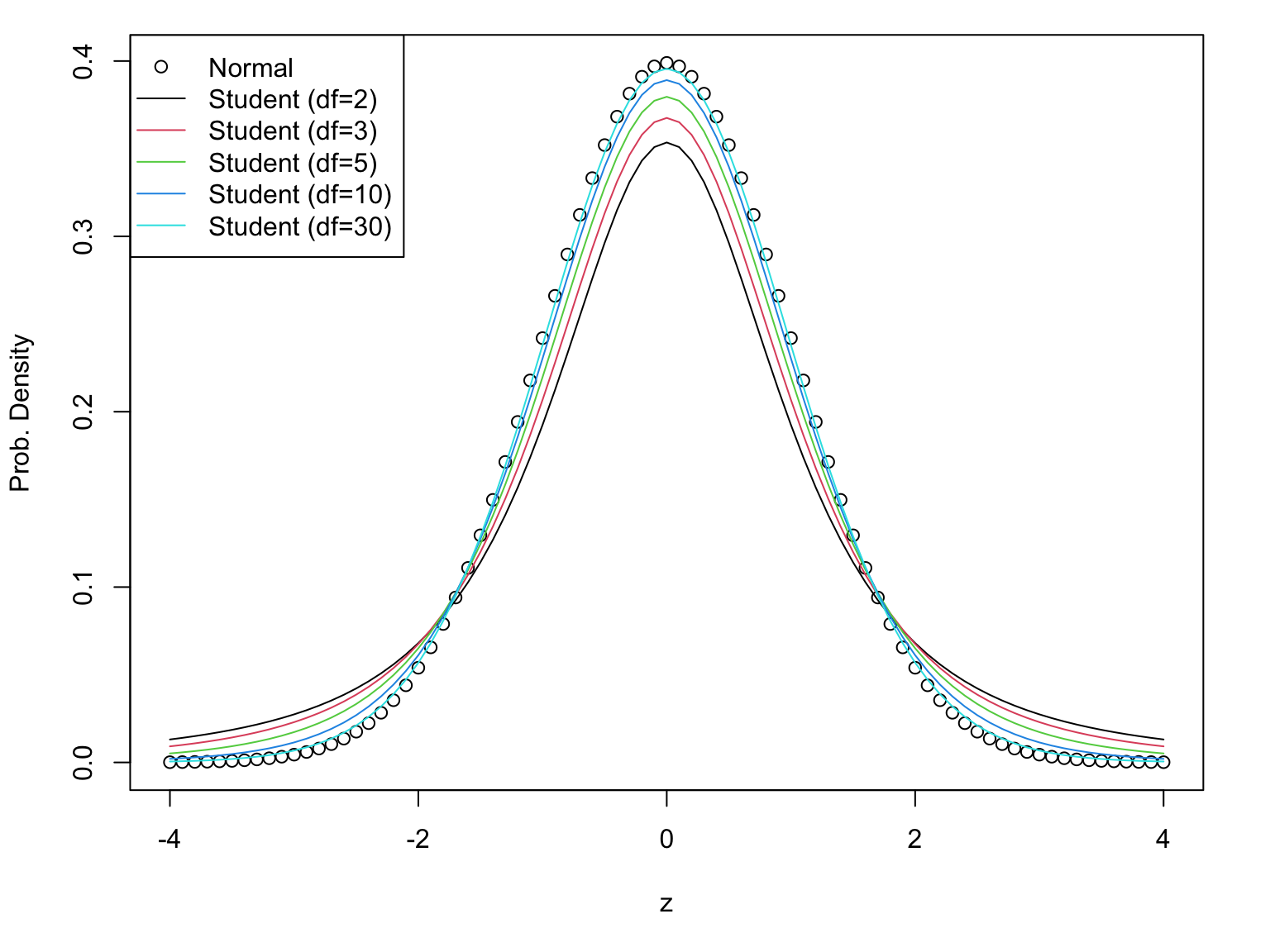# Methodology of Scientific Research

## Sometimes we overgeneralize

This is called “Pareidolia”

🙂

# This is what we want to learn today

## Is the gene differentially expressed?

Let’s say we measured the differential expression of a gene several times

We want to know if the real differential expression is not zero

## Is the gene differentially expressed?

We want to find a confidence interval for the real differential expression

And we want to see if 0 is in the interval

## Assumption: Noise is Normal

We measure the biological signal and noise from the instrument

In general, after normalization, we can assume that the noise follows a normal distribution

If the real expression is $$μ$$, then we measure $X ∼ Normal(μ, σ^2)$

## The average is close to $$μ$$

For each gene we calculate the average $$\bar{X}$$

We know that the average will follow a normal $\bar{X} ∼ Normal(μ, σ^2/n)$ Thus we can make an interval for $$μ$$ $\bar{X}-k⋅σ/\sqrt{n} ≤μ ≤ \bar{X}+k⋅σ/\sqrt{n}$ We have to choose $$k$$ depending on the confidence level we aim

## If we don’t know the distribution

We can always use Chebyshev’s Theorem

k Probability
2 ≥ 1-1/22 = 75%
3 ≥ 1-1/32 = 88.9%
10 ≥ 1-1/102 = 99%
31.6 ≥ 1-1/1000 = 99.9%
k ≥ 1-1/k2

but these intervals are too wide to be useful

## If the population has Normal distribution

In this case we know that the distribution is Normal, so

k Probability
1.959964 95%
2 ≈ 95%
2.5758293 99%
3 ≈ 99%

(These values can be found in tables, or using the computer)

## How to find 𝑘

Take the Normal curve with mean 0 and variance 1

We want the blue area to be large.
So the white area should be small

## Looking for 𝑘

If the blue area is 1-α, then the white area is α

The area of each white part is α/2.
We lool for the points 𝑘 giving areas α/2 and 1-α/2

## Looking for 𝑘

For example, 95% confidence means that 1-α=0.95

Therefore α=0.05, and α/2=0.025

We look for 0.025 and 0.975 in the table

qnorm(0.025)
 -1.959964
qnorm(0.975)
 1.959964

## We don’t know $$σ$$

Until now we have assumed that we knew the population standard deviation

But we do not

We can approximate it with the sample standard deviation

But we have to pay a cost

## The price to pay: Student’s t distribution

This one depends on the degrees of freedom## Student’s t has fat tails

The price to pay for not knowing the population variance is to use Student’s t instead of Normal distribution.

Intervals using Student’s t are wider (and less useful)

To avoid this problem, and get an useful results, we need large enough samples

## Finding 𝑘

k (df=2) k (df=5) k (df=10) Normal Probability
4.3 2.57 2.23 1.96 95%
9.92 4.03 3.17 2.58 99%
31.6 6.87 4.59 3.29 99.9%

## Application

Here we have the measured differential gene expression of several genes

Replica 1 Replica 2 Replica 3
-0.6356720 0.5445543 0.5056405
0.9198619 -0.6887110 -0.2273942
1.1870043 1.0710029 1.3180957
0.1376069 1.7086511 1.1611300
0.8551033 -1.0060231 0.4222059

There are three biological replicas for each gene

## Case 1

The values of first gene are

 -0.6356720  0.5445543  0.5056405

The mean is

 0.1381743

The standard deviation is

 0.6704529

## Interval for Case 1

We have 𝑛=3 values, and we are estimating 1 value (the mean)

Thus, we have 3-1=2 degrees of freedom

The t distribution for 95% and 2 degrees of freedom is

 4.302653

Thus, the 95%-confidence interval for the expression is

 -2.746552  3.022900

The interval contains 0, so it seems that the gene is not differentially expressed

## Case 2

The values of first gene are

 1.187004 1.071003 1.318096

The mean is

 1.192034

The standard deviation is

 0.1236232

## Interval for Case 2

The t distribution for 95% and 2 degrees of freedom is

 4.302653

Thus, the 95%-confidence interval for the expression is

 0.6601268 1.7239418

The interval does not contain 0, so it seems that the gene is differentially expressed

## Redoing at 99% confidence

The t distribution for 99% and 2 degrees of freedom is

 9.924843

Thus, the 99%-confidence interval for the expression is

 -0.03490616  2.41897477

Now the interval contains 0, so it seems that the gene is not differentially expressed

## What is the “good” confidence level?

In Case 2 we have different results depending on the confidence level

One can ask “What is the largest confidence level that will not include 0?”

In other words, what is the smallest α that will not include 0?

That is the 𝑝-value

## Calculating the 𝑝-value

The interval can be written as $-k⋅sd(X)/\sqrt{n} ≤ \bar{X} - μ ≤ k⋅sd(X)/\sqrt{n}$ In the limit case we have $\bar{X}-μ = k⋅sd(X)/\sqrt{n}$ so $k=\frac{\bar{X}-μ}{sd(X)/\sqrt{n}}$

## Looking for 𝑘

In this case we have n=3, mean=1.192 and sd=0.124, so $k=\frac{1.192}{0.124/\sqrt{3}} = 5.567$ We use this value to find α in the table

1-pt(mean(X)/sd(X)/sqrt(3), df=3-1)
 0.01539187

The best confidence level is 1-α=0.985